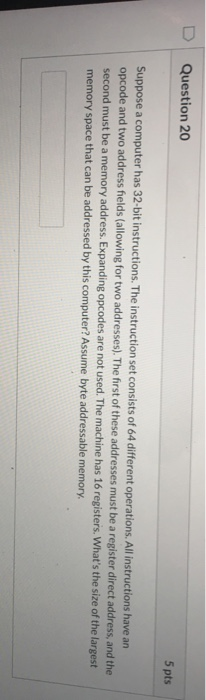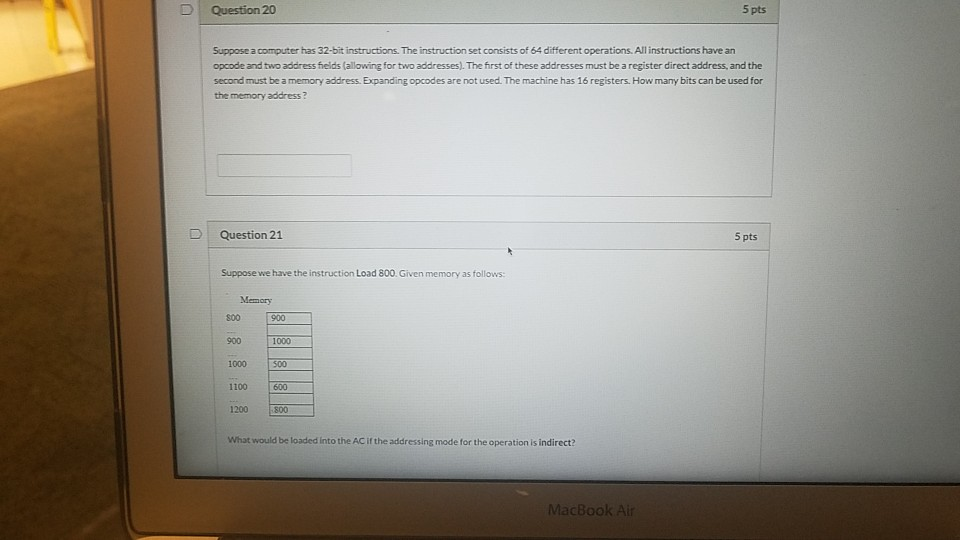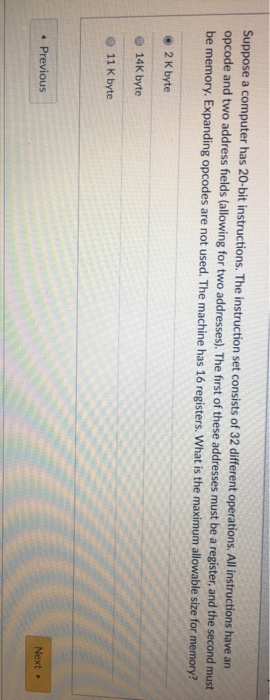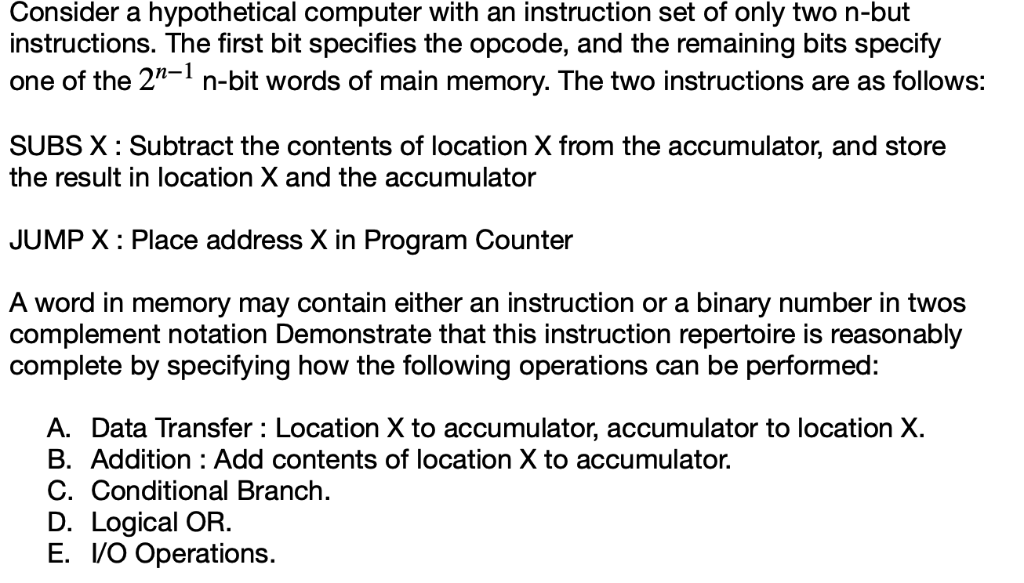Question

A digital computer has a memory unit with 24 bits per word. The instruction set consists of 199 different operations. All instructions have an operation code part (opcode) and an address part (allowing for only one address). Each instruction is stored in one word of memory. [8 marks]

1. How many bits are needed for the opcode?
2. How many bits are left for the address part of the instruction?
3. What is the maximum allowable size for memory?
4. What is the largest signed binary number that can be accommodated in one word of memory?

1. bits are needed for the opcode

Number of operations = 199

--------------------- remainder

2| 199

2| 99    1

2|49 1

2| 24    1

2| 12    0

2|6 0

2|3 0

2|1 1

2|0 1

so to store 199 operations in binary format (11000111)

opcode needs = 8 bits

2. bits are left for the address part of the instruction = 24 - 8 = 16 bits

3. maximum allowable size for memory

Total instructions = 199

1 word = 24 bits

maximum memory size = 199*24 = 4776

4. largest signed binary number that can be accommodated in one word of memory

one word of memory = 24 bits

1 bit for sign

largest binary number = 0111 1111 1111 1111 1111 1111

0 to denote positive number in sign bit position

#### Earn Coins

Coins can be redeemed for fabulous gifts.

Similar Homework Help Questions
• ### A digital computer has a memory unit with 24 bits per word. The instruction set consists...

A digital computer has a memory unit with 24 bits per word. The instruction set consists of 199 different operations. All instructions have an operation code part (opcode) and an address part (allowing for only one address). Each instruction is stored in one word of memory. [] How many bits are needed for the opcode? How many bits are left for the address part of the instruction? What is the maximum allowable size for memory? What is the largest signed...

• ### A digital computer has a memory unit with 24 bits per word. The instruction set consists...

A digital computer has a memory unit with 24 bits per word. The instruction set consists of 110 different operations. All instructions have an operation code part (opcode) and an address part (allowing for only one address). Each instruction is stored in one word of memory. (10 points) How many bits are needed for the opcode? How many bits are left for the address part of the instruction? What is the maximum allowable size for memory? What is the largest...

• ### A digital computer has a memory unit with 24 bits per word.

A digital computer has a memory unit with 24 bits per word. the instructions set consists of 150 different operations. all instructions have an operation codepart(opcode) and an address part (allowing for only one address) Each instruction is stored in one word of memory.a. how many bits are needed for the opcodeb. how many bits are left for the address part of the instruction.c. What is the maximum allowable size of memory.d. what is the largest unsigned binary number that...

• ### 31. A A digital computer has a memory unit with 32 bits per word. The instruction...

31. A A digital computer has a memory unit with 32 bits per word. The instruction set consists of 128 different operations. All instructions have an operation code part (opcode) and an address part (allowing for only one address). Each instruction is stored in one word of memory. How many bits are left for the address part of the instruction. 7 bits 17 bits 25 bits 32 bits

• ### 2. Suppose that a 16M X 16 main memory is built using 512K X 8 RAM...

2. Suppose that a 16M X 16 main memory is built using 512K X 8 RAM chips and memory is word addressable. a) How many RAM chips are necessary? ______ b) How many RAM chips are needed for each memory word? _______ c) How many address bits are needed for each RAM chip? _______ d) How many address bits are needed for all memory? _______ A digital computer has a memory unit with 24 bits per word. The instruction set...

• ### Question 20 5 pts Suppose a computer has 32-bit instructions. The instruction set consists of 64...Question 20 5 pts Suppose a computer has 32-bit instructions. The instruction set consists of 64 different operations. All instructions have an opcode and two address fields (allowing for two addresses). The first of these addresses must be a register direct address, and the second must be a memory address. Expanding opcodes are not used. The machine has 16 registers. What's the size of the largest memory space that can be addressed by this computer?Assume byte addressable memory.

• ### pls both ans Question 20 5 pts Suppose a computer has 32-bit instructions. The instruction set...pls both ans Question 20 5 pts Suppose a computer has 32-bit instructions. The instruction set consists of 64 different operations. All instructions have an opcode and two address fields (allowing for two addresses). The first of these addresses must be a register direct address, and the second must be a memory address. Expanding opcodes are not used. The machine has 16 registers. How many bits can be used for the memory address? Question 21 5 pts Suppose we have...

• ### Suppose a computer has 20-bit instructions. The instruction set consists of 32 different operations. All instructions have an opcode and two address fields (allowing for two addresses). The frst...Suppose a computer has 20-bit instructions. The instruction set consists of 32 different operations. All instructions have an opcode and two address fields (allowing for two addresses). The frst of these addresses must be a register, and the second must be memory. Expanding opcodes are not used. The machine has 16 registers. What is the maximum allowable size for memory? o 2 K byte O 14K byte O 11 K byte Next » ous Suppose a computer has 20-bit instructions....

• ### The memory unit of a computer has 512K words of has an instruction format with 4...The memory unit of a computer has 512K words of has an instruction format with 4 fields: opcode, add and memory address. Given that there are 4 addressing mode, 32-bit instruction, answer the following questions: 32 bits each. The computer ress mode, register address 50 register and 1. How large is the opcode? 2. How large is the address mode field? 3. How large is the register field? 4. How large is the memory address field?

• ### Consider a hypothetical computer with an instruction set of only two n-but instructions. The firs...Consider a hypothetical computer with an instruction set of only two n-but instructions. The first bit specifies the opcode, and the remaining bits specify one of the 2-1 n-bit words of main memory. The two instructions are as follows: SUBS X: Subtract the contents of location X from the accumulator, and store the result in location X and the accumulator JUMP X: Place address X in Program Counter A word in memory may contain either an instruction or a binary...